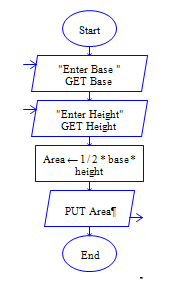# C Program to find area of Right angled triangle

Get length and width of right angled trianlgle and find area of right angled triangle

Sample Input 1:

4 5

Sample Output 1:

10

#### Flow Chart DesignStrongly recommended to Solve it on your own, Don't directly go to the solution given below.

#include<stdio.h> int main() { //write your code here }

#### Program or Solution

``` #include<stdio.h> int main() { int length,width,area; printf("Enter length and width of a triangle:"); scanf("%d %d",&length,&width); area=(length*width)/2; printf("\nArea of Right angled Triangle: %d",area); return 0; } ```

#### Program Explanation

Get length and width of a right angled triangle (using scanf statement)

Calculate area by dividing product of length and width by 2 (area = length * width/2) print area (using printf statement)